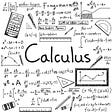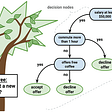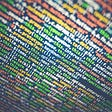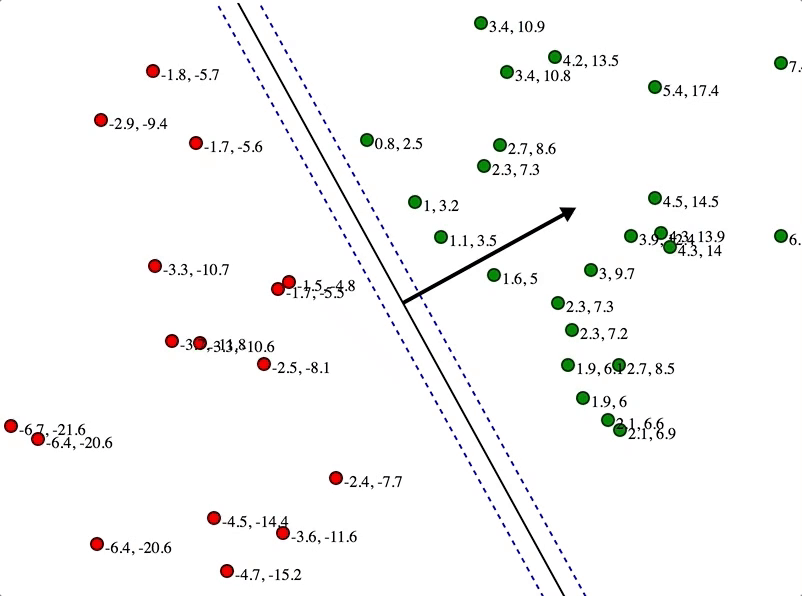# Understanding The Concept Behind Accuracy

As machine learning practitioners, we tend to use accuracy to evaluate the model that we create. But did you know what the concept behind it is? In this article, I would like to share the secret behind it. I will try to write in very easy language so everyone with and without a technical background can understand easily.

# What is Accuracy?

Before going to Rome, at least we need a target to come to that place first. Target always follows with something. -=-= Just a mess intermezzo

Before going all way down, did you know accuracy? Every time I learn about it, I realise that I still know little. As far as I know, in machine learning, accuracy is a concept when a model can predict a domain with the same result as the actual label.

In Machine Learning Course from Google, Accuracy has the following definition.

For example, we have 100 data, consisting of 55 images of oranges and 45 images of apples. Our model predicts that the data consist of 60 images of oranges and 40 images of apples. We could understand that the model has been wrong 10 times and correct 90 times. So the accuracy is like this.

Yeah, the model has 90% accuracy. Maybe the example was too easy to understand. Did you already understand the concept behind it? If not, keep reading this until the end of the article.

# Confusion Matrix

A confusion matrix is another metric to evaluate our classification model. Just like the name, this metric is represented in a matrix form. This matrix gives a comparison between actual and predicted values. I will try to explain the confusion matrix using the image below.

Let’s break the image using the combination of basic math and chemistry; if a positive atom meets another positive will be True, and it has a positive in it (True Positive). If a negative atom meets positive will be False, but there is a positive in it (False Positives). If a positive atom meets another negative will be False, but it has a negative in it (False Negatives). Finally, when a negative atom meets another negative will be True, and it has a negative in it (True Negatives).

Using this matrix, we could understand many insights into other metrics, such as accuracy, precision, recall, and f1-score. To make the article specific, this article will focus on accuracy only.

# Confusion Matrix in Binary Classification

Binary classification is a concept of classifying things in binary format (0 and 1), or it is easier to say that it’s a two-class classification. The easiest way to explain this is by using an example.

Okay, let’s say we have 10 images consisting of 6 dogs and 4 cats. Because it’s a binary classification, let’s say cat as 0 and dog as 1. Then our model predicted there would be 7 dogs and 3 cats.

`ACTUAL = [1,0,1,1,0,0,1,1,0,1]PREDICTION = [1,1,1,1,1,0,0,1,1,0]`

From the data above, we can say that the classification is not good but not bad. Nah, accuracy is a metric score to count how accurate a model gives a prediction based on actual data. The confusion matrix gives a clearer insight into how we got the accuracy.

`# Import necessary libraryfrom sklearn.metrics import confusion_matriximport matplotlib.pyplot as pltimport seaborn as sns# Create confusion matrixmatrix = confusion_matrix(ACTUAL, PREDICTION, labels=[0,1])# Visualize the confusion matrix cm = sns.heatmap(matrix, annot=True, cmap="Blues")cm.set_title("Confusion Matrix", fontsize=25)cm.set_xlabel("Actual Label", fontsize=20)cm.set_ylabel("Predicted Label", fontsize=20)plt.show()`

The output of the code above will be like this.

But let me give some notes in the picture to make it more understandable.

Based on the image from Confusion Matrix, we could say that:

• Cell 1 = True Positives → 1 (1 Picture is a cat and predicted as a cat)
• Cell 2 = False Positives → 3 (3 Picture is a dog but predicted as a cat)
• Cell 3 = False Negatives → 2 (2 Picture is a cat but predicted as a dog)
• Cell 4 = True Negatives → 4 (4 Picture is a dog and predicted as a dog)

The formula to get accuracy is `all correct prediction / all prediction` or, in the confusion matrix, we could say this.

In the example above, we put accuracy based on cat images, so it only has 1 TP, if we use dog images, it will have 4 TP, and since it was a binary classification, it doesn’t matter where you put the data first. Let’s count it.

Yeah, the accuracy is 0.5; if you don’t trust it, you could also check using the library, and the output you will get will be the same.

`# Import necessary libraryfrom sklearn.metrics import accuracy_score# Count the accuracyaccuracy = accuracy_score(ACTUAL, PREDICTION)accuracy=> 0.5`

I think this is enough about binary classification. Let’s move into the Multi-Class classification.

# Confusion Matrix in Multi-Class Classification

Multi-Class classification is a concept of classifying things into more than two classes. To make it easier, let’s think with an example. Imagine we had three classes consisting of apple, orange, and kiwi.

As same as before in this article, I don’t want you to create a model because it is beyond the purpose of this article. Think that we already have the actual and predicted class from a model.

`ACTUAL = ["apple","orange","kiwi","kiwi","apple","apple","orange","kiwi","orange","kiwi","apple","apple","orange","kiwi","apple"]PREDICTION = ["apple","orange","kiwi","kiwi","apple","apple","orange","kiwi","orange","orange","kiwi","apple","apple","orange","kiwi"]`

Let’s build the confusion matrix and plot it.

`# Create the confusion matrixmatrix = confusion_matrix(ACTUAL, PREDICTION, labels=["apple", "orange", "kiwi"])# Visualize the confusion matrix cm = sns.heatmap(matrix, annot=True, cmap="Blues",                 xticklabels=["apple", "orange", "kiwi"],                 yticklabels=["apple", "orange", "kiwi"])cm.set_title("Confusion Matrix", fontsize=25)cm.set_xlabel("Actual Label", fontsize=20)cm.set_ylabel("Predicted Label", fontsize=20)plt.show()`

The output will be like this.

Again please permit me to give some notes to make it clearer.

Because it’s a multiclass classification, the accuracy from each class can be different with all of the data. Based on the terminology, we can count accuracy like this.

You can prove it is using `accuracy_score` and will get the same output

`# Count the accuracyaccuracy = accuracy_score(ACTUAL, PREDICTION)accuracy=> 0.66666666`

Nah, that was the concept of accuracy counted on all data. If you want to know the specific accuracy in every class, you can find it using this concept. For example, think you want to see the accuracy based on the apple class.

Because you are taking the apple class as the target, it will automatically become the True Positive. Then, we can call the horizontal line from it as False Positive and the vertical line from it as False Negative; what about the rest? The rest will automatically become True Negative because it doesn’t refer to apple at all. Finally, we could count the accuracy of the apple class.

If you want to count the accuracy based on the orange class, the concept is the same, just put the True Positive in the middle of the confusion matrix, like this.

The accuracy will be like this.

Did you get the concept? Can you implement it in the last class? Well, let’s do it together.

If you asked, how can True Positive be in the below square? The answer is that it doesn’t matter at the position; the focus is on the data target you choose. If we choose kiwi, automatically, cell 9 will be the True Positives, and the rest of the data beyond the horizontal and vertical lines will be True Negatives. The accuracy from a class of Kiwis is like this.

From the tree accuracy above, we can understand our model is not good at predicting kiwi, so we can focus on improving accuracy.

Did you get it? If you are in doubt, why is it necessary to count the accuracy based on every class, not all at once, because it is a great idea to improve your model by understanding the data also, you can also get other metrics such as precision, f1-score, specificity, etc. Finally, you will get better accuracy by focusing on a class that worsens your classification model.

# Conclusion

Congratulations on reading this article this way. Let me review this article, accuracy is a metric that is commonly used in model prediction. We can also get accuracy by counting the confusion matrix despite using an accuracy score. I hope you understand the story behind classification very clearly right now. Thanks for reading.

 Bharathi, Confusion Matrix for Multi-Class Classification (2021), Analytics Vidhya

 Normalized Nerd, Machine Learning with Scikit-Learn Python | Accuracy, F1 Score, Confusion Matrix, YouTube

 My Notebook, Metrics Accuracy and Confusion Matrix, Kaggle

--

--

--

## More from Data Folks Indonesia

Artikel tentang seluruh yang berkaitan dengan data mulai dari machine learning, data analisis, data engineering, data science, business intelligence

## Experimenting with XGBoost## Data Project — Pokemon Battle Prediction (2): Predict with Machine Learning## Intel MLSL Makes Distributed Training with MXNet Faster## Kickstarter — Predictive Analytics## Machine Learning Explained## The Matrix Calculus You Need For Deep Learning (Notes from a paper by Terence Parr and Jeremy…## Go Face Recognition Tutorial — Part 1## Semi Supervised learning for YOLOv4 using pseudo labeling## Handhika Yanuar Pratama

A Stoic Developer || Junior Data Engineer || Dreamer

## Decision tree algorithm for classification: A basic introduction with example in python## Pruning in Decision Trees## How does CNN provide a computer with vision?## Support Vector Machine(SVM) explained